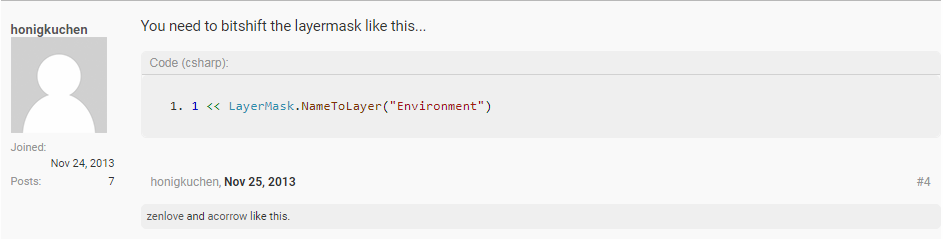# OverlapCircleNonAlloc Layermask in Unity not working and the way to Fix it

Hey all,

I ran into this issue when I was trying to use OverlapCircleNonAlloc to find enemies within distance of each other for targeting.  It stumped me for about an hour until I came across this page.  At the bottom a very straight forward direction was left and it ended up working, pictured below.After some research in the documentation here I understand the left shift operator in C# a little better also known as bit shift!  Upfront I will let you know that there is also a right-shift operator (`>>`).

The left-shift operator (`<<`) shifts its first operand left by the number of bits specified by its second operand. The type of the second operand must be an int or a type that has a predefined implicit numeric conversion to `int`. -Microsoft

In other words, << shifts bits over in a byte.  For example if an int is 32 bits and looks like this '00000000000000000000000000000001' and you use this shift '1 << 8' you move the 1 in that int over 8 times to look like this '00000000000000000000000100000000'.

What happens here is each movement on the bit to the left it doubles itself until it is a 256 (1 to 2 to 4 to 8 to 16 to 32 to 64 to 128 to 256) in the 8th position.  The logic is that Layers use an integer bit mask and there are 32 possible layers (check unity editor, starts at 0 and ends at 31).

So to simplify, if a bit is a 0 or a 1 and all Layers reside within a 32 bit integer, the way to find your layer within those 32 bits is to use a bit shift to find your layer.